# POWER Function – Raise to a Power in Excel, VBA, & G Sheets

This Tutorial demonstrates how to use the Excel POWER Function in Excel to calculate the number raised to a power.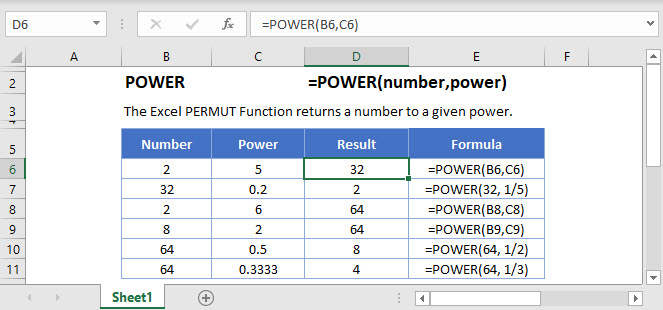## POWER Function Overview

The POWER Function Calculates a number raised to a power.

To use the POWER  Excel Worksheet Function, select a cell and type: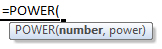(Notice how the formula inputs appear)

## POWER Function Syntax and Inputs:

number – A number.

power – The exponent number.

## POWER Function

The POWER Function can raise a number to a power or an exponent.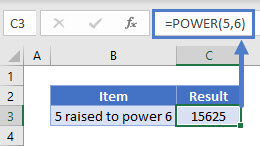Notice here the formula raises the number 5 to the sixth power.

## POWER Function – Squared/Cubed

The POWER Function can return a square or cube of a number.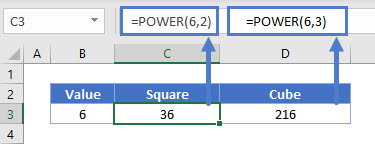This example raises the number to its second (squared) or third (cubed) power.

## POWER Function – Square Root

The POWER Function can return the square root of a number.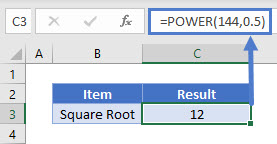Square root of a number is a value, when multiplied by itself, returns the number. The SQRT Function can also be used, click here to learn more.

## POWER Function – Cube Root

The POWER Function can also calculate the cube root of a number.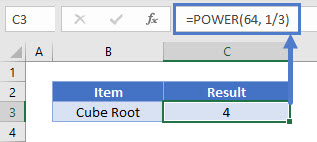The Cube Root of a number is the same as that number raised to one-third (⅓) power.

The POWER Function works exactly the same in Google Sheets as in Excel: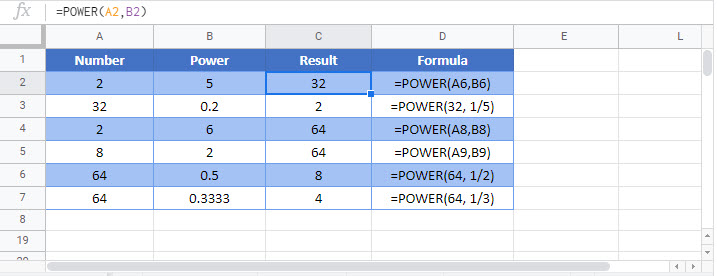Use the POWER Function to raise a number to a specified power.

`=power(2,4) is the same as 2^4`

## POWER Examples in VBA

You can also use the POWER function in VBA. Type:
`application.worksheetfunction.power(number,power)`
For the function arguments (number, etc.), you can either enter them directly into the function, or define variables to use instead.

The statementy above will return 2^3  = 8

Or, we can use variables as parameters of our VBA function: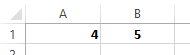Will return 4^5 = 1024